# 1. (Coding problem) Consider the satellite equations GMx (x2 + y2)3/2' GMY (x2 + y2)3/2" with...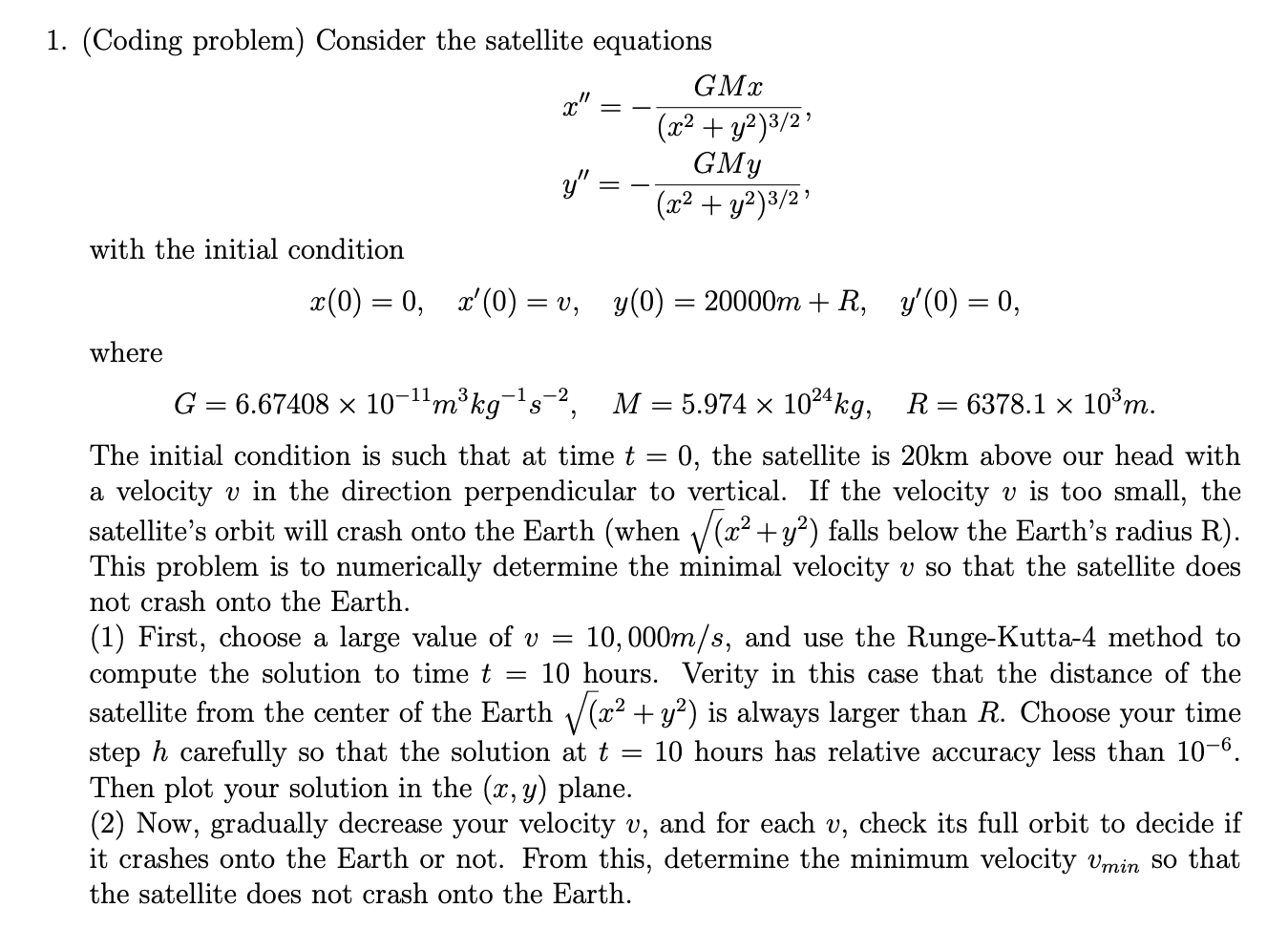1. (Coding problem) Consider the satellite equations GMx (x2 + y2)3/2' GMY (x2 + y2)3/2" with the initial condition x(0) = 0, x'(0) = v, y(0) = 20000m + R, y'(0) = 0, where G = 6.67408 x 10-11mºkg-15-2, M = 5.974 x 1024 kg, R= 6378.1 x 10°m. The initial condition is such that at time t = 0, the satellite is 20km above our head with a velocity v in the direction perpendicular to vertical. If the velocity v is too small, the satellite's orbit will crash onto the Earth (when (x2 + y2) falls below the Earth’s radius R). This problem is to numerically determine the minimal velocity v so that the satellite does not crash onto the Earth. (1) First, choose a large value of v = 10,000m/s, and use the Runge-Kutta-4 method to compute the solution to time t = 10 hours. Verity in this case that the distance of the satellite from the center of the Earth V (x2 + y2) is always larger than R. Choose your time step h carefully so that the solution at t = 10 hours has relative accuracy less than 10-6. Then plot your solution in the (x,y) plane. (2) Now, gradually decrease your velocity v, and for each v, check its full orbit to decide if it crashes onto the Earth or not. From this, determine the minimum velocity Vmin so that the satellite does not crash onto the Earth.

(1) The system can be first converted into a system of four ordinary differential equations and then can be solved using ode45 (Runke-Kutta 4th order) of MATLAB by the following code:

G=6.67408*10^(-11);
M=5.974*10^(24);
R=6378.1*10^3;
v=10000;
f = @(t,x) [x(2);(-G*M*x(1))/((x(1))^2+(x(3))^2)^(3/2);x(4);(-G*M*x(1))/((x(3))^2+(x(3))^2)^(3/2)]; % The system %
[t,xa] = ode45(f,[0 10],[0 v 20000+R 0]);
distance=sqrt((xa(85,1))^2+(xa(85,3))^2) % distance of the satelite from the centre of earth %

plot(xa(:,1),xa(:,3))
xlabel('x(t)'), ylabel('y(t)')

You can see the distance we get is 6398880 which is larger than R, i.e., 6378100. The plot (x,y) is given below :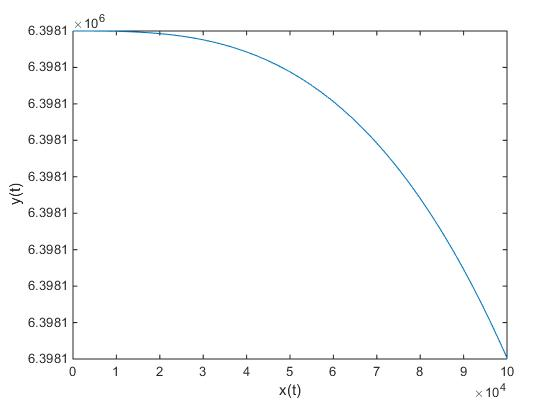(b) To determine the v_{min}, one can change v in the above MATLAB code and check the distance for each of this v. Then see for which it is less than R.

#### Earn Coin

Coins can be redeemed for fabulous gifts.

Similar Homework Help Questions
• ### 2. The velocity field for a fluid is defined by u = [y/(x2 + y2)] and...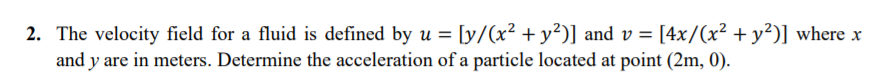2. The velocity field for a fluid is defined by u = [y/(x2 + y2)] and v = [4x/(x2 + y2)] where x and y are in meters. Determine the acceleration of a particle located at point (2m, 0).

• ### All of 10 questions, please. 1. Find and classify all the critical points of the function. f(x,y) - x2(y - 2) - y2 » 2. Evaluate the integral. 3. Determine the volume of the solid that is inside the...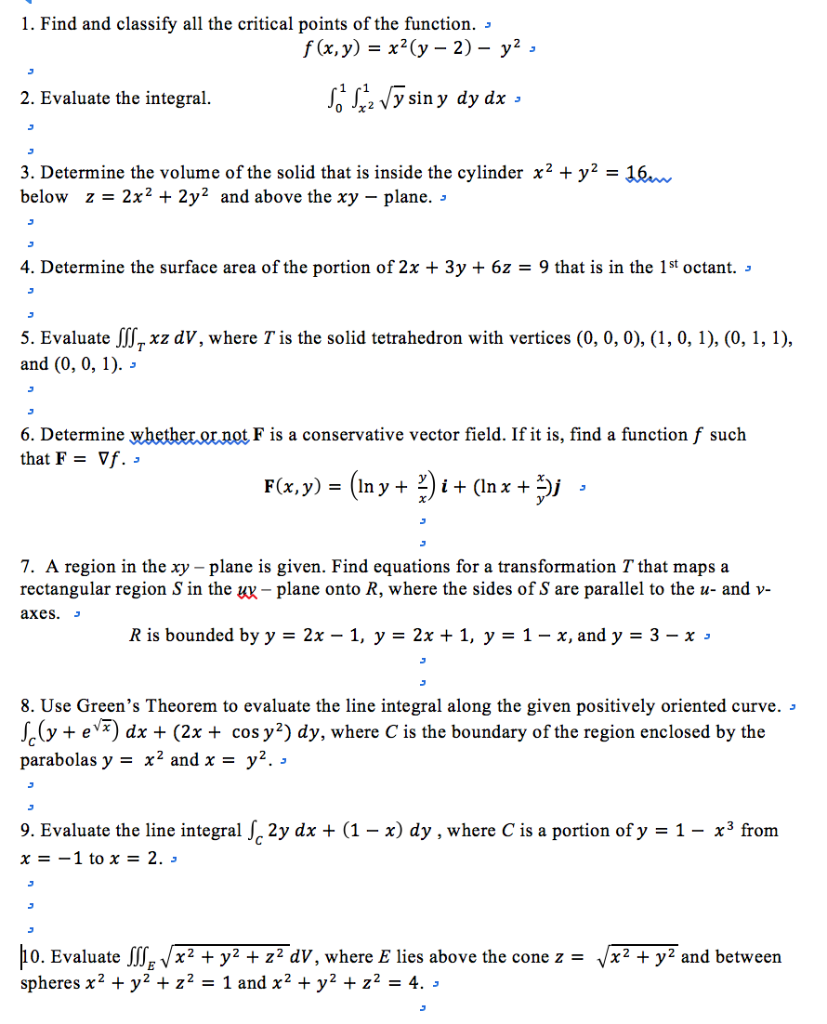All of 10 questions, please. 1. Find and classify all the critical points of the function. f(x,y) - x2(y - 2) - y2 » 2. Evaluate the integral. 3. Determine the volume of the solid that is inside the cylinder x2 + y2- 16 below z-2x2 + 2y2 and above the xy - plane. 4. Determine the surface area of the portion of 2x + 3y + 6z - 9 that is in the 1st octant. » 5. Evaluate JSxz...

• ### Question 3. Consider the function h: R3 → R h(x, y, 2) = (x2 + y2...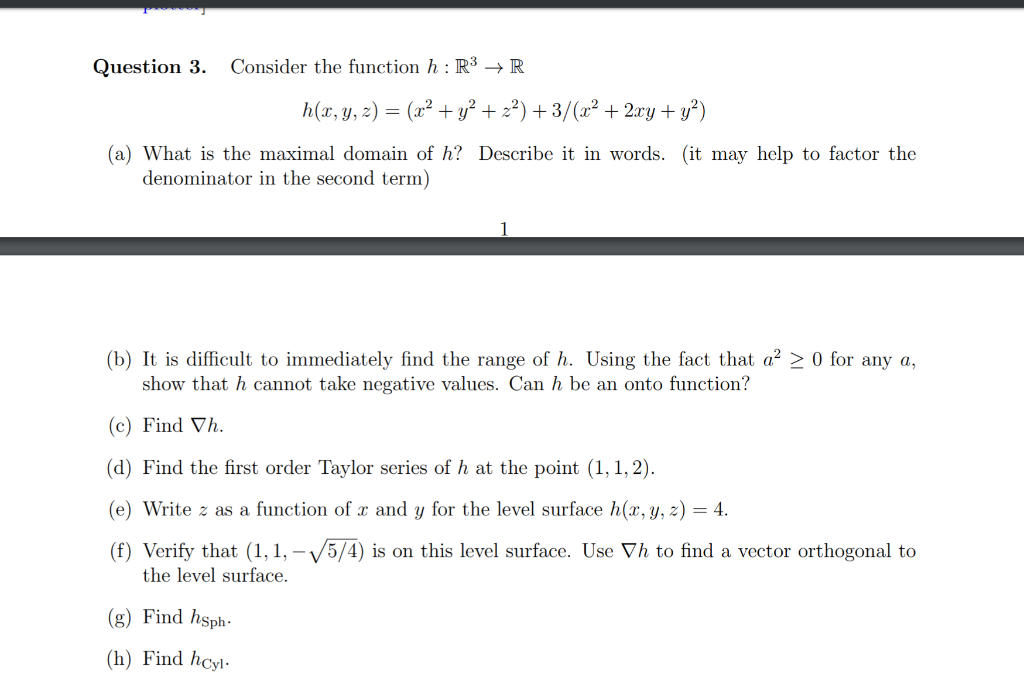Question 3. Consider the function h: R3 → R h(x, y, 2) = (x2 + y2 + 2) +3/(x2 + 2xy + y) (a) What is the maximal domain of h? Describe it in words. (it may help to factor the denominator in the second term) > 0 for any a, (b) It is difficult to immediately find the range of h. Using the fact that a show that h cannot take negative values. Can h be an onto function?...

• ### 6. (4 pts) Consider the double integral∫R(x2+y)dA=∫10∫y−y(x2+y)dxdy+∫√21∫√2−y2−√2−y2(x2+y)dxdy.(a) Sketch the region of integration R in Figure 3.(b)...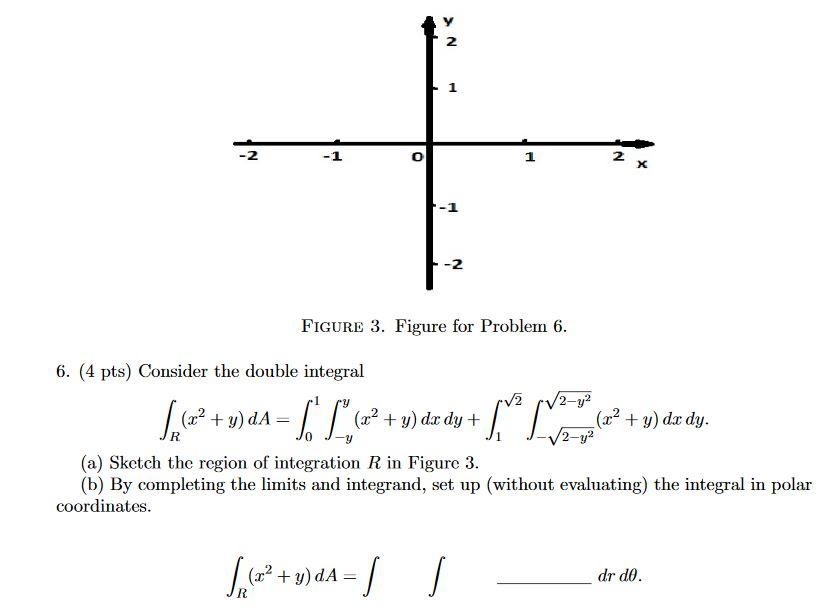6. (4 pts) Consider the double integral∫R(x2+y)dA=∫10∫y−y(x2+y)dxdy+∫√21∫√2−y2−√2−y2(x2+y)dxdy.(a) Sketch the region of integration R in Figure 3.(b) By completing the limits and integrand, set up (without evaluating) the integral in polar coordinates. 2 1 2 X -2 FIGURE 3. Figure for Problem 6. 6. (4 pts) Consider the double integral V2 2-y2 (2? + y) dA= (32 + y) dx dy + (x2 + y) dx dy. 2-y? (a) ketch the region of integration R in Figure 3. (b) By completing...

• ### Solve the separable initial value problem. tan(sin(x^(2) 1. y' = 2x cos(x2)(1 + y2), y(0) =...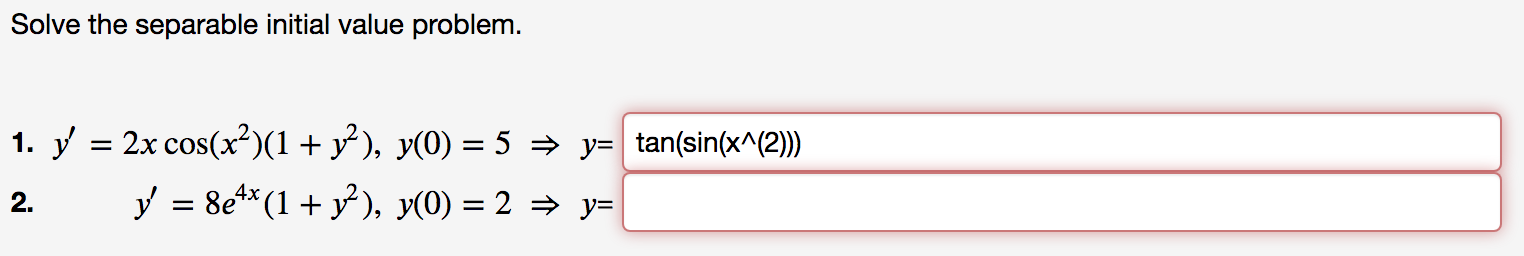Solve the separable initial value problem. tan(sin(x^(2) 1. y' = 2x cos(x2)(1 + y2), y(0) = 5 → y= 2. v' = 8e4x(1 + y2), y(0) = 2 + y=

• ### Previous Problem Problem List Next Problem x2 + y2 with 2 2 0; its boundary (1...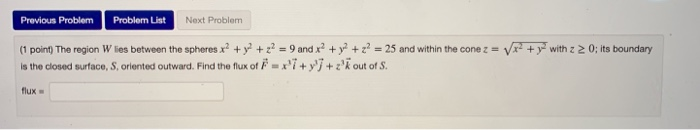Previous Problem Problem List Next Problem x2 + y2 with 2 2 0; its boundary (1 point) The region W lies between the spheres x2 + y2 + z = 9 and x2 + y2 + z = 25 and within the cone z = Is the closed surface, S., oriented outward. Find the flux of F = x +y +z'k out of S. flux

• ### 9. The upper half of the ellipsoid tr + ty? + Z2-1 intersects the cylinder x2 + y2-y 0 in a curves C. Calculate tfe circulation of v y'i+y+3i k around C by using Stokes Theorem. x2 + y2 inter...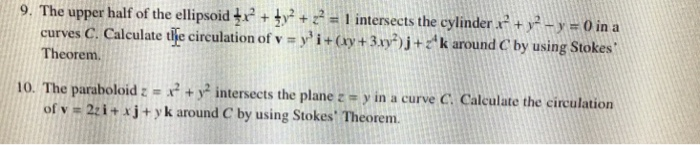9. The upper half of the ellipsoid tr + ty? + Z2-1 intersects the cylinder x2 + y2-y 0 in a curves C. Calculate tfe circulation of v y'i+y+3i k around C by using Stokes Theorem. x2 + y2 intersec ts the plane z y in a curve C. Calculate the circulation 10. The paraboloid z of v 2zi+ x j + y k around C by using Stokes Theorem. 9. The upper half of the ellipsoid tr + ty?...

• ### Problem 1.20. Let f(z, y)-(X2-y2)/(z2 + y2) 2 for x, y E (0, 1]. Prove that...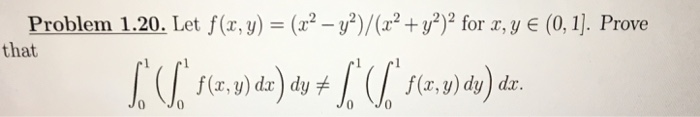Problem 1.20. Let f(z, y)-(X2-y2)/(z2 + y2) 2 for x, y E (0, 1]. Prove that f(x, y) dx dy f f(x,y) dy)dr. Jo Jo JoJo

• ### 6. (4 pts) Consider the double integral∫R(x2+y)dA=∫10∫y−y(x2+y)dxdy+∫√21∫√2−y2−√2−y2(x2+y)dxdy.(a) Sketch the region of integration R in Figure 3.(b)...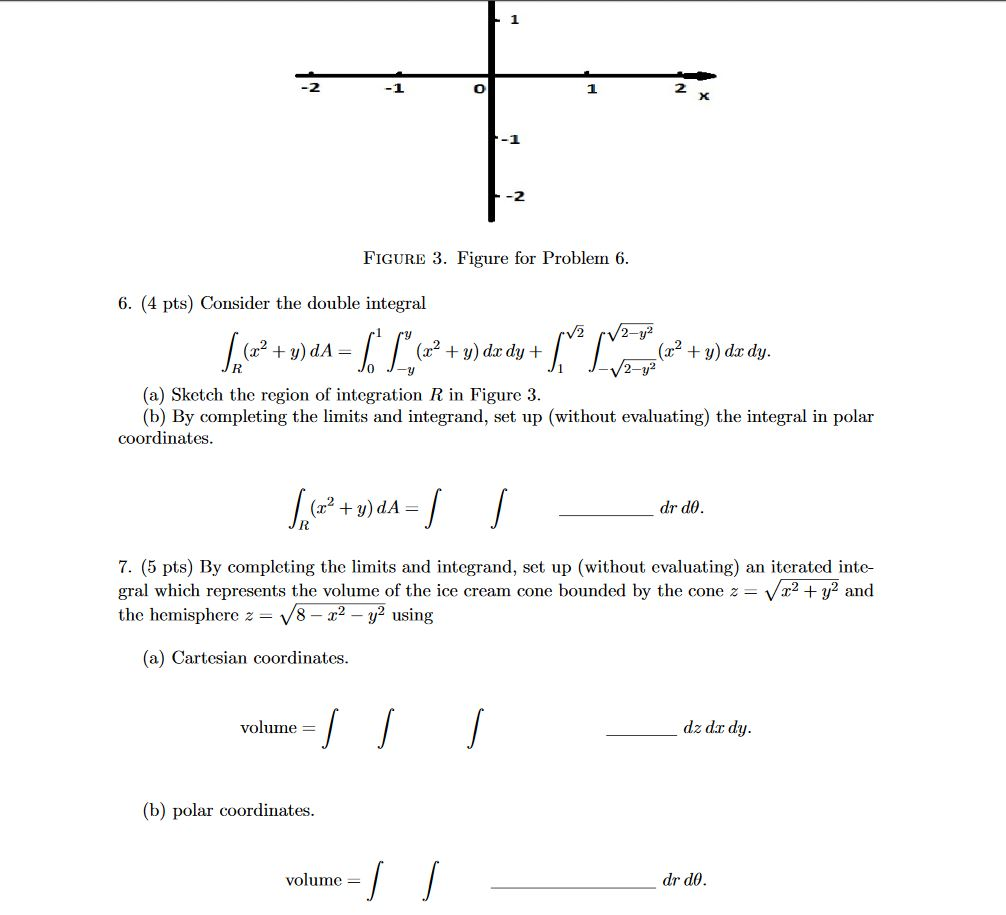6. (4 pts) Consider the double integral∫R(x2+y)dA=∫10∫y−y(x2+y)dxdy+∫√21∫√2−y2−√2−y2(x2+y)dxdy.(a) Sketch the region of integration R in Figure 3.(b) By completing the limits and integrand, set up (without evaluating) the integral in polar coordinates.∫R(x2+y)dA=∫∫drdθ.7. (5 pts) By completing the limits and integrand, set up (without evaluating) an iterated inte-gral which represents the volume of the ice cream cone bounded by the cone z=√x2+y2andthe hemisphere z=√8−x2−y2using(a) Cartesian coordinates.volume =∫∫∫dz dxdy.(b) polar coordinates.volume =∫∫drdθ. -1 -2 FIGURE 3. Figure for Problem 6. 6. (4 pts)...

• ### Question 1. Consider these real-valued functions of two variables: T In (x2 + y2) (a) () What is ...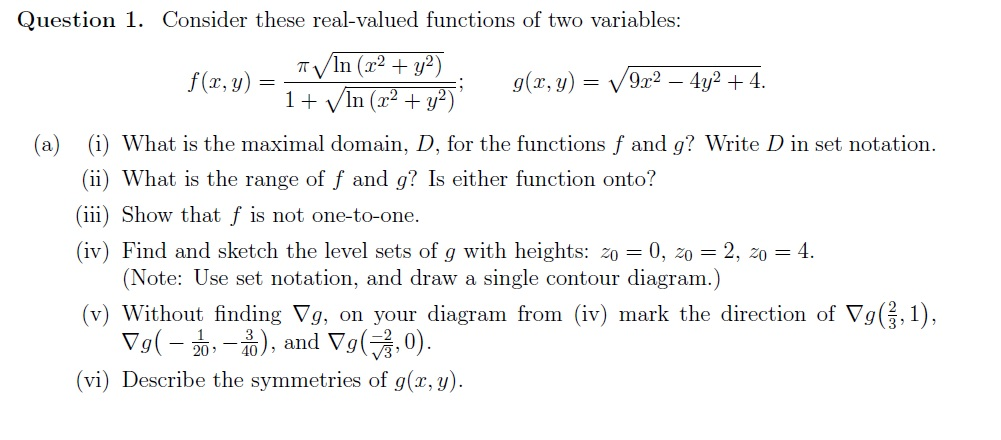Question 1. Consider these real-valued functions of two variables: T In (x2 + y2) (a) () What is the maximal domain, D, for the functions f and g? Write D in set notation. (ii) What is the range of f and g? Is either function onto? ii) Show that f is not one-to-one. (iv) Find and sketch the level sets of g with heights: z00, 2, 04 (Note: Use set notation, and draw a single contour diagram.) (v) Without finding...